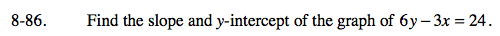### Home > CAAC > Chapter 8 > Lesson 8.2.5 > Problem8-86

8-86.Solve the equation for y in order to put it into Slope-Intercept (y = mx + b) form.

6y = 3x + 24

y = 0.5x + 4

slope = 0.5, y-intercept = (0, 4)